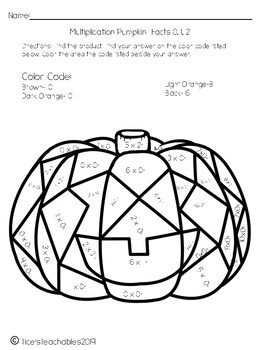# Color by Code Multiplication PumpkinsSubject
Resource Type
File Type

PDF

(4 MB)
Product Rating
Standards
• Product Description
• StandardsNEW

Beginners multiplication color by code pumpkin.

There are two worksheets and the multiplication facts that are needed to complete the pumpkins are 0,1,2, 10, and 11.

Your students will have a great time completing this fall activity while practicing their multiplication.

It would work great in a math center as an independent activity.

3.OA.C.7

3.OA.A.1

Fluently multiply and divide within 100, using strategies such as the relationship between multiplication and division (e.g., knowing that 8 × 5 = 40, one knows 40 ÷ 5 = 8) or properties of operations. By the end of Grade 3, know from memory all products of two one-digit numbers.
Interpret products of whole numbers, e.g., interpret 5 × 7 as the total number of objects in 5 groups of 7 objects each. For example, describe a context in which a total number of objects can be expressed as 5 × 7.
Total Pages
N/A
N/A
Teaching Duration
N/A
Report this Resource to TpT
Reported resources will be reviewed by our team. Report this resource to let us know if this resource violates TpT’s content guidelines.